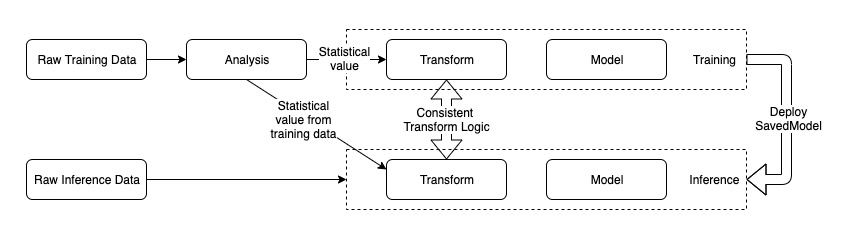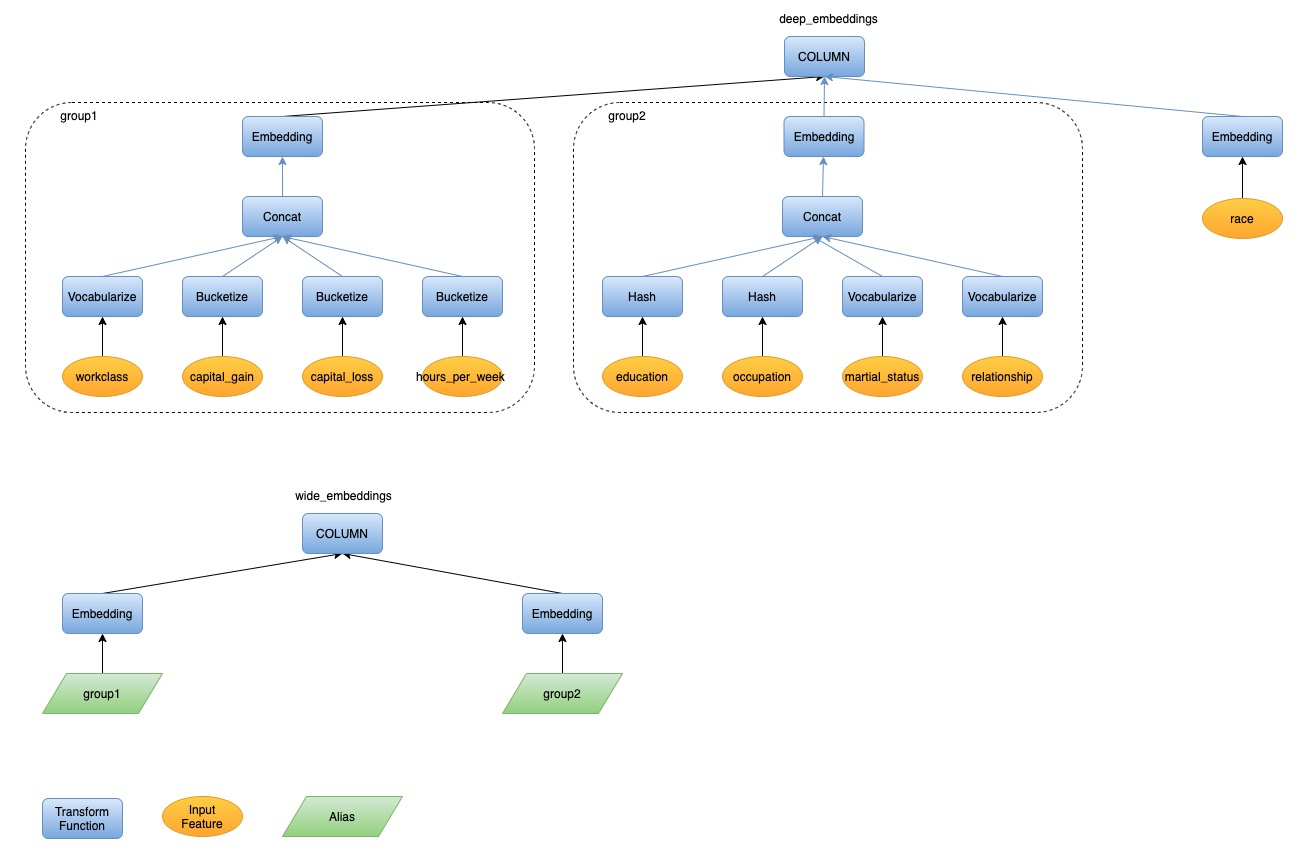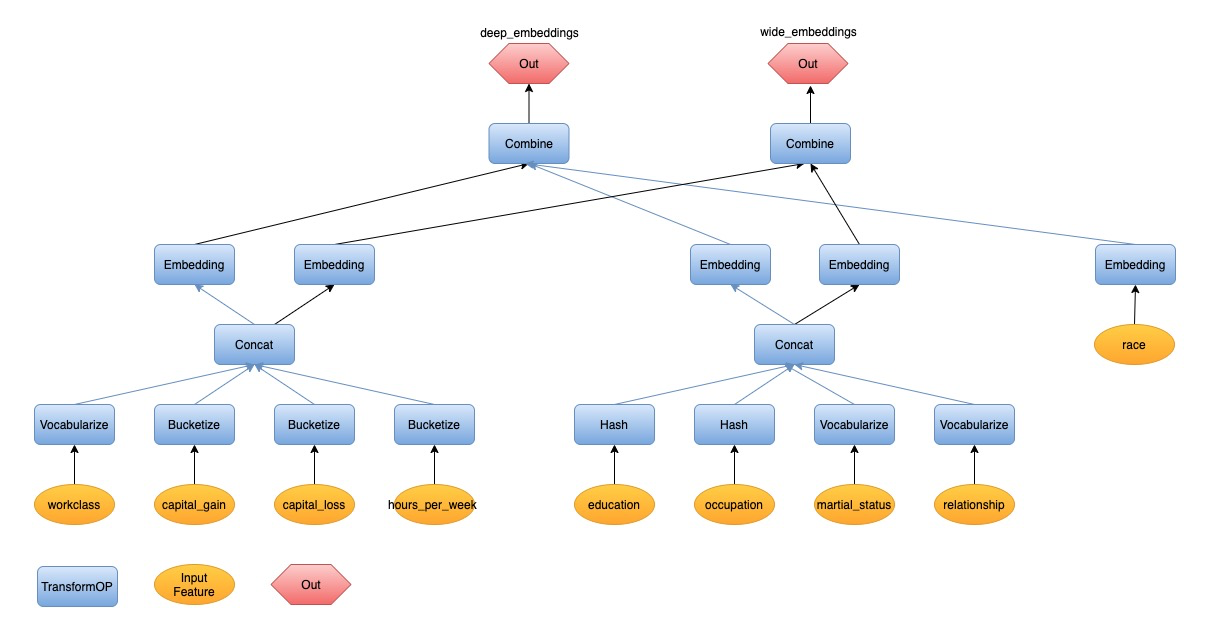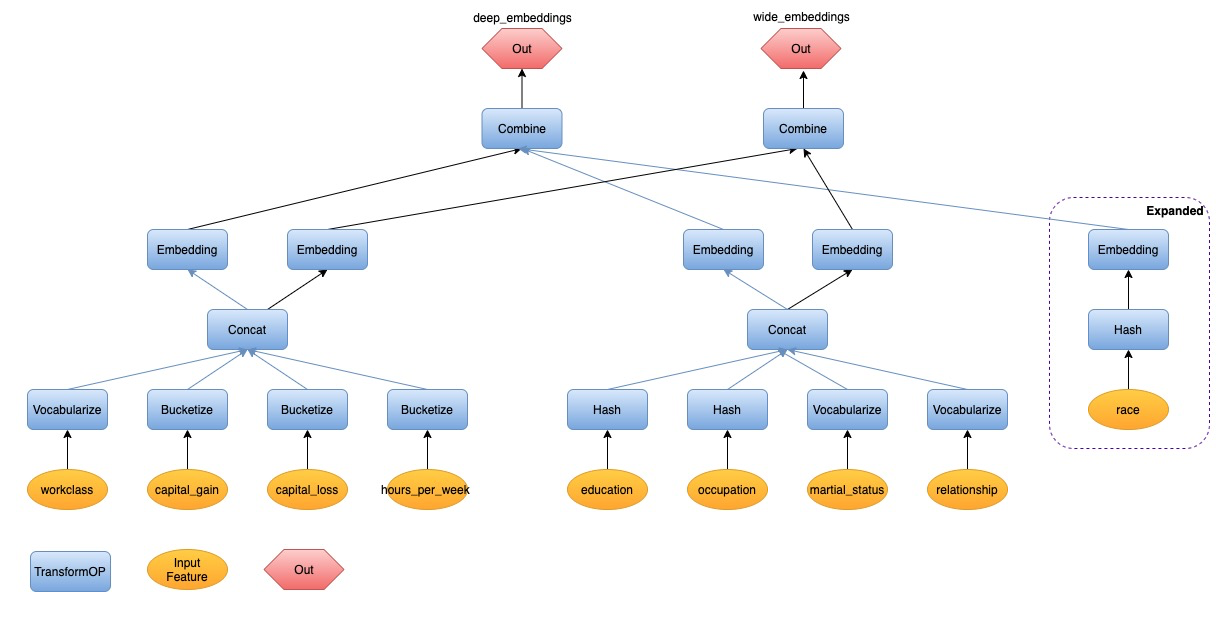# Data Analysis and Tranformation

## The Problem

We want to add data analysis, in addition to data transformation, into the end-to-end machine learning pipeline generated by SQLFlow.

End-to-end machine learning means a pipeline from raw data to model training and applications. With SQLFlow, we propose to describe complex pipelines with SQL in concisely. An essential part of the solution is to describe the processing of raw data into model inputs. SQLFlow provides a COLUMN clause for this purpose.

Currently, SQLFlow converts content in the `COLUMN` clause into the Python source code of data transformation. The following example would call the Feature Column API `categorical_column_with_hash_bucket` from TensorFlow to convert a string `address` from the database into a single-element tensor of an integer ID.

``````SELECT * FROM welfare TO
TRAIN DNNRegressor
LABEL income;
``````

However, data transform often requires parameters. The above example requires the bucket size, 1000. Other examples include

``````standardize(age, mean, stdev)
normalize(size, min, max)
categorize(city, city_name_list)
``````

The challenge is – users don’t want to specify these parameters; instead, they want SQLFlow to do the data statistics and derives these parameters from data automatically. So, the above examples become

``````standardize(age)
normalize(size)
categorize(city)
``````

In the terminology of TFX Transform, the word analysis refers to data statistics that derives the above parameters, given which, the transforming refers to the conversion of raw data into model inputs. This design document is about making SQLFlow support data analysis, in addition to transformation.

## The Syntax Extension

Without any syntax extension, users can write SQL statements for data analysis. For example, the following SQL statement works with MySQL and can normalize the field `size` of table `plates`.

``````SELECT 1.00 * (t1.size - t2.size_min) / t2.size_range
FROM plates t1
JOIN
(
SELECT
MIN(size) AS size_min,
MAX(size) - MIN(size) AS size_range
FROM plates
) t2
ON 1 = 1
``````

Unfortunately, the above code is tricky and hard to read. And users might have to write it multiples time – one before training and one before the prediction – thus doubles the source code complexity.

For the goal of making deep learning more straightforward, we hope our users can write the following statement.

``````SELECT * FROM plates
TO TRAIN DNNRegressor
COLUMN normalize(size)
LABEL price
INTO a_model;
``````

This syntax implies that SQLFlow can convert the above statement into a Python program that does data analysis and data transformation.

To use the trained model to make predictions, we hope the users don’t have to rewrite the COLUMN clause. For example, the following statement assumes that the table `new_plates` has a field `size`. The converted Python code should normalize `size` before sending it to the input of `a_mode`.

``````SELECT * FROM new_plates TO PREDICT price USING a_model;
``````

If the user doesn’t want normalization, but the raw value, as the input, she could write the following.

``````SELECT * FROM new_plates TO PREDICT price USING a_model COLUMN size
``````

Or, if she wants standardization instead of normalization, she could write the following.

``````SELECT * FROM new_plates
TO PREDICT price
USING a_model
COLUMN standardize(size)
``````

How if the table new_plates doesn’t have the field used to train the model? How if there is no field named `size`, but a field `diameter`. We should allow users to use the latter as well.

``````SELECT * FROM new_plates
TO PREDICT price
USING a_model
COLUM normalize(diameter)
``````

Please be aware that if the user wants normalization, she must write `normalize(diameter)` instead of `diameter`. The following example uses the raw value of `diameter` without normalization.

``````SELECT * FROM new_plates TO PREDICT price USING a_model COLUMN diameter
``````

## The Challenge

From the above examples, we see that a challenge is that the `TO TRAIN` clause must be able to save the input field names and the data analysis results and transformation steps together with the model, so to make sure that the predictions using the same data transformation as the training.

### TensorFlow Transform

TensorFlow Transform is the open source solution for data transform in TensorFlow Extended. Users need write a Python function `preprocess_fn` to define the preprocess logic. The preprocessing function contains two groups of API calls: TensorFlow Transform Analyzers and TensorFlow Ops. Analyzer will do the statistical work on the training dataset once and convert to constant tensors. And then the statistical value and TensorFlow Ops will make the concrete transform logic as a TensorFlow graph and then convert the data record one by one. The graph will be used for both training and serving. Let’s take normalizing (min-max normalization) the column value `capital_gain` in census income dataset for example. The following is the `preprocess_fn` definition with TensorFlow Transform:

``````import tensorflow_transform as tft

def preprocess_fn(inputs):
outputs = inputs.copy()
outputs["capital_gain"] = tft.scale_to_0_1(inputs["capital_gain"])
return outputs
``````

From users’ perspective, SQLFlow users prefer to write SQL instead of python. It’s not user-friendly if we integrate TF Transform with SQLFlow directly.

## Our Approach

Data transform contains two key parts: analyzer and transformer. Analyzer scans the entire data set and calculates the statistical values such as mean, max, min, etc. Transformer refers the statistical values if any as parameters to build the concrete transform logic. And then it transforms the data records one by one. The transform logic should be consistent between training and inference.From the perspective of SQLFLow, SQL can naturally support statistical work just like the analyzer. Feature column API and keras preprocessing layer can take charge of the transform work as transformer. We plan to use SQL and feature column/keras preprocessing layer together to do the data analysis and transform work.

Since we use SQL to do the analysis work, SQL requires the table schema to be wide - one feature per column. So, we will normalize the table schema at first.

### Normalize Table Schema to Be Wide

Wide table means that it only stores one feature or label in one column. It’s more friendly to SQL for data analysis.

#### Why

Let’s take this analysis work for example: calculate the max of `age` and mean of `education_num`. If it’s a wide table as follows:

age education_num income_category
39 10 0
52 9 1
28 13 0

The SQL statement for analysis is straightforward:

``````SELECT
MAX(age) AS age_max,
AVG(education_num) AS education_num_mean
FROM census_income
``````

Sometimes users may encode multiple feature values as a key-value string and store it in one column of the table, just like the following table:

features income_category
age:39;education_num:10 0
age:52;education_num:9 1
age:28;education_num:13 0

We can’t use SQL directly to do the same analysis work as above.

#### Proposal

We can provide common tools to normalize the table schema. If the data is stored in MaxCompute table, we can use PyODPS and UDF to complete the task. Please look at the doc flatten_odps_key_value_table for the detailed design.

After normalizing the table schema, we can do data analysis and transformation on this normalized table. The preprocess pipeline is described using SQLFlow statement. The logic can be very flexible and the current synatx of COLUMN clause cannot cover all the scenarios, such as standardize `age`. We want to design SQLFlow syntax extension to fully express the analysis and transform process elegantly.

### SQLFlow Syntax Extension

We can extend the SQLFlow syntax and enrich the `COLUMN` expression. We propose to add some built-in functions to describe the transform process. We will implement common used functions at the first stage.

Name Transformation Statitical Parameter Input Type Output Type
NORMALIZE(x) Scale the inputs to the range [0, 1]. `out = x - x_min / (x_max - x_min)` x_min, x_max number (int, float) float64
STANDARDIZE(x) Scale the inputs to z-score subtracts out the mean and divides by standard deviation. `out = x - x_mean / x_stddev` x_mean, x_stddev number float64
BUCKETIZE(x, num_buckets, boundaries) Transform the numeric features into categorical ids using a set of thresholds. boundaries number int64
HASH_BUCKET(x, hash_bucket_size) Map the inputs into a finite number of buckets by hashing. `out_id = Hash(input_feature) % bucket_size` hash_bucket_size string, int32, int64 int64
VOCABULARIZE(x) Map the inputs to integer ids by looking up the vocabulary vocabulary_list string, int32, int64 int64
EMBEDDING(x, dimension) Map the inputs to embedding vectors N/A int32, int64 float32
CROSS(x1, x2, …, xn, hash_bucket_size) Hash(cartesian product of features) % hash_bucket_size N/A string, number int64
CONCAT(x1, x2, …, xn) Concatenate multiple tensors representing categorical ids (zero-based) into one tensor. The output id space is the sum of inputs. N/A int32, int64 int64
COMBINE(x1, x2, …, xn) Combine multiple tensors into one tensor N/A number number

Please check more discussion about `CONCAT` transform function

Let’s take the following SQLFlow statement for example.

``````SELECT *
FROM census_income
TO TRAIN DNNClassifier
WITH model.hidden_units = [10, 20]
COLUMN
NORMALIZE(capital_gain),
STANDARDIZE(age),
EMBEDDING(hours_per_week, dimension=32)
LABEL label
``````

It trains a DNN model to classify someone’s income level using the census income dataset. The transform expression is ```COLUMN NORMALIZE(capital_gain), STANDARDIZE(age), EMBEDDING(hours_per_week, dimension=32)```. It will normalize the column capital_gain, standardize the column age, and then map hours_per_week to an embedding vector.

Next, Let’s see a more complicated scenario. The following SQL statment trains a wide and deep model using the same dataset.

``````SELECT *
FROM census_income
TO TRAIN WideAndDeepClassifier
COLUMN
COMBINE(
EMBEDDING(
CONCAT(
VOCABULARIZE(workclass),
BUCKETIZE(capital_gain, num_buckets=5),
BUCKETIZE(capital_loss, num_buckets=5),
BUCKETIZE(hours_per_week, num_buckets=6)
) AS group_1,
8),
EMBEDDING(
CONCAT(
HASH(education),
HASH(occupation),
VOCABULARIZE(martial_status),
VOCABULARIZE(relationship)
) AS group_2,
8),
EMBEDDING(race, 8)
) FOR deep_embeddings,
COMBINE(
EMBEDDING(group1, 1),
EMBEDDING(group2, 1)
) FOR wide_embeddings
LABEL label
``````

SQLFlow will compile the `COLUMN` expression to Python code of data transformation. Let’s take the SQLFlow statement above for example to describe the compilation workflow step by step in the next section.

### COLUMN Expression Compilation Workflow

#### Parse COLUMN Expression into AST

Given a COLUMN expression, syntax parsing is naturally the first step. We will parse one COLUMN expression to one AST. For the SQL statement containing two column expressions above, we will parse them into two ASTs. Please check the following figure of the AST for the example SQL.#### Convert the AST into a DAG of Transform Flow

To convert ASTs into a transform flow, we will do an one-to-one mapping at first.

1. Transform Function(AST) to TransformOP(Transform Flow). Each TransformOP has parameters. Some parameters can be gotten directly from the parsed result of COLUMN clause. And some parameters need be derived from data analysis and we will talk about it in detail in the following steps.
2. Input Feature(AST) to Input Feature(Transform Flow).
3. Column Node(AST) to Combine + Out(Transform Flow). We may have two or more column expressions, one column for one AST (tree structure). If we combine these ASTs, we will get a forest at this time.

We may also have alias with keyword `AS` just like `AS group1` in the example SQL. The alias stands for a part of transform flow which is shared by multiple upstream nodes. And then the forest will become a DAG.#### Expand the TransformOP

Let’s focus on the clause `EMBEDDING(race, 8)` in the example SQL. As we know, the SQLFlow statement is intention driven. We tell it to do embedding for the input feature `race` directly and don’t tell how to map it into an integer id at first. And the latter is required for the model built using native TensorFlow. So we will expand the `Embedding` TransformOP and add `Hash` to do the id conversion work. The DAG will be expaneded from `race -> Embedding` to `race -> Hash -> Embedding`, just like the following graph.At this time, we have gotten a complete graph of the transform flow. But some TransformOP don’t have all the required parameters, such as `vocabulary list` for `VOCABULARIZE(workclass)`. We will do the data analysis work to derive the parameter value.

#### Derive the Parameters of TransformOP from Data Analysis

SQLFlow will generate the analysis SQL to calculate the statistical value. Please check the following analysis SQL for each transform function.

NORMALIZE(x)

``````SELECT
MIN(x) AS x_min,
MAX(x) AS x_max
FROM data_table;
``````

STANDARDIZE(x)

``````SELECT
AVG(x) AS x_mean,
STDDEV(x) AS x_stddev
FROM data_table;
``````

BUCKETIZE(x, num_buckets=5)

``````SELECT
PERCENTILE(age, ARRAY(0.2, 0.4, 0.6, 0.8)) AS x_bucket_boundaries
FROM data_table;
``````

VOCABULARIZE(x)

``````SELECT DISTINCT(x)
FROM data_table;
``````

HASH(x)

``````SELECT (COUNT(DISTINCT(x)) * 3) AS x_hash_bucket_size
FROM data_table;
``````

Note: If we assign COUNT(DISTINCT(x)) as hash_bucket_size, the conflict ratio is high - about 40%. So we multiply it with a factor `3` here. And the factor is tunable here.

#### Derive the parameters of TransformOP from DAG Walkthrough

Some parameters of TransformOP can’t be gotten from data analysis. For example, `Embedding` needs the parameter `input_dimension`, it’s equals to `num_buckets` attribute of `CONCAT` which is the dependency of `Embedding`. In one sentence, we need walk through the DAG and calculate the parameters of one TransformOP from its dependency.

#### Generate the Transform Python code from the DAG

Now we have a complete DAG: a complete Graph describing the dependency of TransformOPs and each TranformOP has all the required parameters. We can make typological sort on the DAG and get an ordered list. Then we generate the python code according to this order. A TransformOP node will generate a line of python code. A line of python code is an api call to feature column or keras preprocessing layers from TensorFlow native API and our extensions in elasticdl_preprocessing. Please check the sample code generated from the example SQL: keras preprocessing layer version and feature column version.

At this moment, we have gotten the full transform code and can prepare for model training. For the sample SQL, we will combine the transform code and `WideAndDeepClassifier` from model zoo to the submitter program. The bridge between these two parts is important for the combination.

#### The Bridge Between Transform Code and Model Definition from Model Zoo

The model definition in the model zoo is a python function or a python class. It has some input parameters. The transform python code is built upon feature column api or keras preprocessing layers. The bridge between transform code and model definition should cover the transform logic using both feature column and keras preprocessing layers.

Tensors is a good choice for the bridge. The keyword `FOR` in the column clause means that it will output one tensor, and two `FOR` keywords output two tensors. So the COLUMN expressions in example SQL output two tensors: `deep_embeddings` and `wide_embeddings`.

For keras functional model, the python function of the model is `wide_and_deep_classifier`. The names of the output tensors match the names of the input parameters in the function. We will combine the transform code and model definition through parameter binding according to the name.

For keras subclass model, it has a core method `def call(self, inputs)` to describe the forward pass logic. But we cannot get how many tensors the model accepts from the parameter `inputs`. To solve this problem, we propose to add a decorator `@declare_model_inputs` on the model class to inject some metadata. The metadata tells us the number and names of the input tensor for this model.

``````@declare_model_inputs("wide_embeddings", "deep_embeddings")
class WideAndDeepClassifier(tf.keras.Model):
def __init__(self):
pass

def call(self, inputs):
pass
``````

And then we can get the input tensor names `[wide_embeddings, deep_embeddings]` from the static attribute of the model class - `WideAndDeepClassifier._model_inputs`. Model zoo service can load the python module and get the metadata using this way. It’s useful for the parameter binding between COLUMN clause and model definition.

And when do we use this decorator during model development? Please check the following:

• If ML specialist develops the model structure and verify it using `model.fit`, the decorator is not necessary.
• If ML specialist run the machine learning pipeline locally using SQLFlow / run model unit test using SQLFlow / submit model into SQLFlow model zoo, ML specialist need add the decorator.

## Further Consideration

From the syntax design above, we can see that the COLUMN clause can be very long. It’s will be challenging to users to write the entire COLUMN clause if there are hundreds of features or even more from source table. And it’s common in the search and recommendatation scenario. In the long term, user can write simpiflied COLUMN clause or even don’t need write it. SQLFlow will do feature deriviation and auto-complete the COLUMN clause.